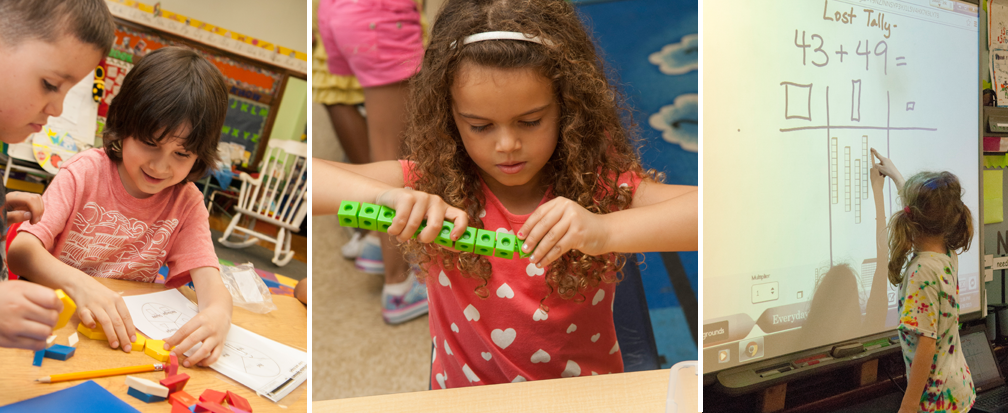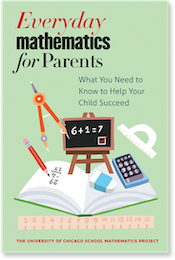Everyday Mathematics third-edition Grade Level Goals have been revised to align with the Common Core State Standards for Mathematics (CCSS-M), which were widely adopted in 2010. Both versions of the curriculum's goals are available below. For more information on standards, please see Meeting Standards with Everyday Mathematics.

### Common Core State Standards (CCSS) Edition Grade Level Goals

Expand All | Collapse All
Number and Numeration
Understand the Meanings, Uses, and Representations of Numbers Rote counting Verbally count in sequence to 10 and beyond; develop flexibility in counting, including counting on and counting backward.
Rational counting Count objects with one-to-one correspondence and know the last counting word tells "how many."
Place value and notation Develop an awareness of numbers and their uses; associate number names, quantities, and written numerals; recognize and use different ways to represent numbers (for example, groups of objects or dots).
Understand Common Numerical Relations Comparing and ordering numbers Compare and order groups of objects using words such as more, fewer, less, same.
Operations and Computation
Understand Meanings of Operations Models for the operations Solve and create number stories using concrete modeling; explore part-whole relationships (for example, 5 is made of 2 and 3).
Data and Chance
Select and Create Appropriate Graphical Representations of Collected or Given Data Data collection and representation Collect and represent data in a variety of ways, focusing on concrete and pictorial representations.
Analyze and Interpret Data Data analysis Use graphs to answer simple questions.
Measurement and Reference Frames
Understand the Systems and Processes of Measurement; Use Appropriate Techniques, Tools, Units, and Formulas in Making Measurements. Length, weight, and angles Distinguish and describe size attributes, including length, weight, and capacity or volume; compare objects according to various size attributes.
Understand the Systems and Processes of Measurement; Use Appropriate Techniques, Tools, Units, and Formulas in Making Measurements Units and systems of measurement Become familiar with standard measuring tools and their uses; begin to understand the concept of measurement units and the idea that measurement can be quantified.
Time Sequence familiar events in time.
Geometry
Investigate Characteristics and Properties of Two- and Three-Dimensional Geometric Shapes Plane and solid figures Recognize and describe basic 2-dimensional geometric shapes; explore the relationships between basic 2-dimensional and 3-dimensional shapes.
Apply Transformations and Symmetry in Geometric Situations Transformations and symmetry Recognize and describe the position and location of objects; use spatial reasoning in concrete tasks, such as putting together puzzles and creating collages and block structures.
Patterns, Functions, and Algebra
Understand Patterns and Functions Patterns and functions Recognize and match attributes of objects, such as size, shape, and color; use rules to sort objects; use rules to create and extend repeating patterns.

### 3rd Edition Grade Level Goals

Expand All | Collapse All
Number and Numeration
Understand the Meanings, Uses, and Representations of Numbers Rote counting Verbally count in sequence to 10 and beyond; develop flexibility in counting, including counting on and counting backward.
Rational counting Count objects with one-to-one correspondence and know the last counting word tells "how many."
Place value and notation Develop an awareness of numbers and their uses; association number names, quantities, and written numerals; recognize and use different ways to represent numbers (for example, groups of object or dots). )
Understand Common Numerical Relations Comparing and ordering numbers Compare and order groups of objects using words such as more, fewer, less, same.
Operations and Computation
Understand Meanings of Operations Models for the operations Solve and create number stories using concrete modeling; explore part-whole relationships (for example, 5 is made of 2 and 3).
Data and Chance
Select and Create Appropriate Graphical Representations of Collected or Given Data Data collection and representation Collect and represent data in a variety of ways focusing on concrete and pictoral representations.
Analyze and Interpret Data Data analysis Use graphs to answer simple questions.
Measurement and Reference Frames
Understand the Systems and Processes of Measurement; Use Appropriate Techniques, Tools, Units, and Formulas in Making Measurements Length, weight, and angles Distinguish and describe size attributes, including length, weight, and capacity or volume; compare objects according to various size attributes.
Units and systems of measurement Become familiar with standard measuring tools and their uses; begin to understand the concept of measurement units and the idea that measurement can be quantified.
Time Sequence familiar events in time
Geometry
Investigate Characteristics and Properties of Two- and Three-Dimensional Geometric Shapes Plane and solid figures Recognize and describe basic 2-dimensional geometric shapes; explore the relationships between basic 2-dimensional and 3-dimensional shapes.
Apply Transformations and Symmetry in Geometric Situations Transformations and symmetry Recognize and describe the position and location of objects; use spatial reasoning in concrete tasks, such as putting together puzzles and creating collages and block structures.
Patterns, Functions, and Algebra
Understand Patterns and Functions Patterns and functions Recognize and match attributes of objects, such as size, shape, and color; use rules to sort objects; use rules to create and extend repeating patterns.The University of Chicago School Mathematics Project

University of Chicago Press